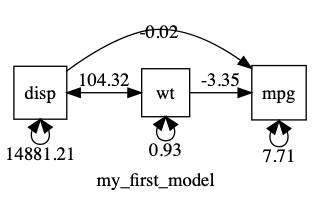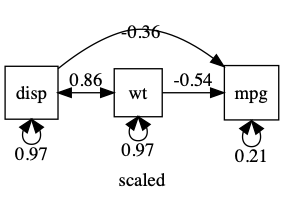# Overview

This post covers `umx_residualize`, `umx_scale`, `umx_rename`

There are a range of cases where it is useful to manipulate data for modeling: for convenience (e.g. re-naming variables), to help ensure good solutions, e.g., by re-scaling variables, scaling specialized data e.g. twin data. This post covers `umx` support for these needs.

### umx_residualize

A common need is to residualize variables prior to modeling. You might, for instance, want to control for (residualize) the effects of age on depression scores.

This post covers:

1. Simple residualization using `umx_residualize`
2. Residualizing twin (wide) data using `umx_residualize`

We often want to residualize several variables prior to analysis. In twin-data, it is critical to use the same residual formula for all copies of a variable in the wide dataset. This can lead to complex, error-prone and lengthy code. For instance, this is how one might think to residualize two variables in base-R:

#### Simpler tasks: a formula interface to residualization

`R` has great support for linear modeling with an insightful formula interface. Getting residuals can still benefit from a helper, however.

Here’s residualization using base `R`: Here, you need to remember to set the `na.action` to “na.exclude”, and then do the residualization as a second step after the modelling.

``````m1 = lm(mpg ~ cyl + disp, data = mtcars, na.action = na.exclude)
r2\$mpg = residuals(m1)

``````

Compare this to the same kind of thing done using `umx_residualize`:

``````r1 = umx_residualize(mpg ~ cyl + I(cyl^2) + disp, data = mtcars)

``````

### Wide Data

A common data format for `umx` is wide: 1-family per row for twin data. This complex-ifies normal approaches to residualization.

You MUST residualize data for both twins using the same beta weights. This means making the data long, doing the model, get the residualization results, then setting the data back to wide format.

``````twinData\$MPQAchievement_T1 <- residuals(lm(Achievement_T1 ~ Sex_T1 + Age_T1 + I(Age_T1^2), data = twinData, na.action = na.exclude))
twinData\$MPQAchievement_T2 <- residuals(lm(Achievement_T2 ~ Sex_T2 + Age_T2 + I(Age_T2^2), data = twinData, na.action = na.exclude))
``````

One complex line of code for each twin, perhaps repeated for 10 more variables, generating 20-lines of complex code… Lot's of opportunity for a tupo ☺

You also have to remember to `na.exclude` your `lm`() call.

But more than this: the residualization separately on twin 1 and twin 2 is a massive error: different betas are applied to the variable for twin 1 and twin 2. We would need to make the data long, generate betas for all family members, then take the data back out to wide. A pain.

With `umx_residualise` this can be reduced in two ways. This one-line residualizes both twin’s data, and doesn’t require typing all the suffixes:

``````twinData = umx_residualize(Achievement ~ Sex + Age + I(Age^2), suffix = "_T", data = twinData)
``````

`umx_residualise` can also residualize more than one dependent variable (though not with formulae yet). So this works:

``````twinData = umx_residualize(c("Achievement", "Motivation"), c("Sex", "Age"), suffix = "_T", data = twinData)
``````

`umx_residualize` does this in one line:

``````df= umx_residualize(var="DEP", covs="age", suffixes= c("_T1", "_T2"), data=df)
``````

### umx_scale

As usual, the post assumes you’ve loaded `umx`:

``````library("umx")
``````

Starting with our very simple model of three raw variables:

``````m1 = umxRAM("my_first_model", data = mtcars,
umxPath(cov = c("disp", "wt")),
umxPath(c("disp", "wt"), to = "mpg"),
umxPath(v.m. = c("disp", "wt", "mpg"))
)
``````

If we `plot` this, we can see that displacement has a MUCH bigger variance than the other variables…

`````` plot(m1, mean = FALSE)
``````Having variances differ by orders of magnitude can make it hard for the optimizer. In such cases, you can often get better results making variables more comparable: in this case, for instance, by converting disp (with its units of cubic inches) into displacement in litres. This will keep the variance of displacement smaller, and closer to that of the other variables.

``````df = mtcars
df\$engine_litres = .016 * df\$disp
m1 <- umxRAM("scaled", data = df,
umxPath(cov = c("engine_litres", "wt")),
umxPath(c("engine_litres", "wt"), to = "mpg"),
umxPath(v.m.   = c("engine_litres", "wt", "mpg"))
)
``````

`plot(m1, mean=FALSE)`A common workflow is to standardize all variables. note: Plot can give you a standardized output: just say` std=TRUE`

``````df = umx_scale(mtcars)
m1 <- umxRAM("scaled", data = df,
umxPath(cov = c("disp", "wt")),
umxPath(c("disp", "wt"), to = "mpg"),
umxPath(v.m.   = c("disp", "wt", "mpg"))
)
plot(m1, mean=FALSE)

```````umxAPA(std=TRUE)` will also standardize many types of `lm`, `glm` etc.

### Renaming variables

Above, in the process of getting a variable with smaller variance, we created the less cryptic “engine_litres” variable name. `umx` provides `umx_rename` to ease this more generally.

``````df = umx_scale(mtcars)
df = umx_rename(df, old=c("disp", "wt"), replace=c("engine_displacement", "car_weight"))

m1 <- umxRAM("scaled", data = df,
umxPath(cov = c("engine_displacement", "car_weight")),
umxPath(c("engine_displacement", "car_weight"), to = "mpg"),
umxPath(v.m.   = c("engine_displacement", "car_weight", "mpg"))
)

plot(m1, std=TRUE, mean = FALSE)
``````1. TODO: A tutorial on data simulation with `umx_make_TwinData`, `umx_make_fake_data`, and `umx_make_MR_data`